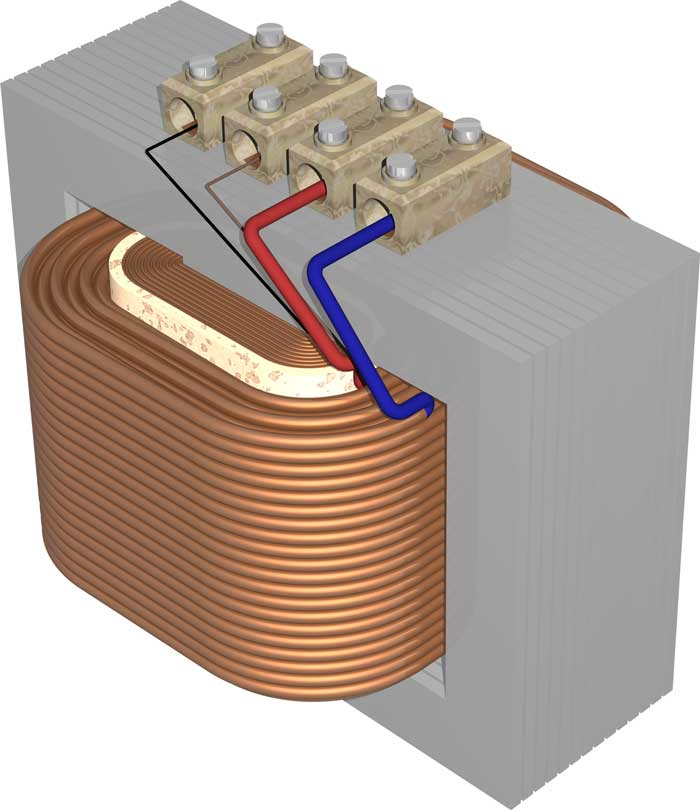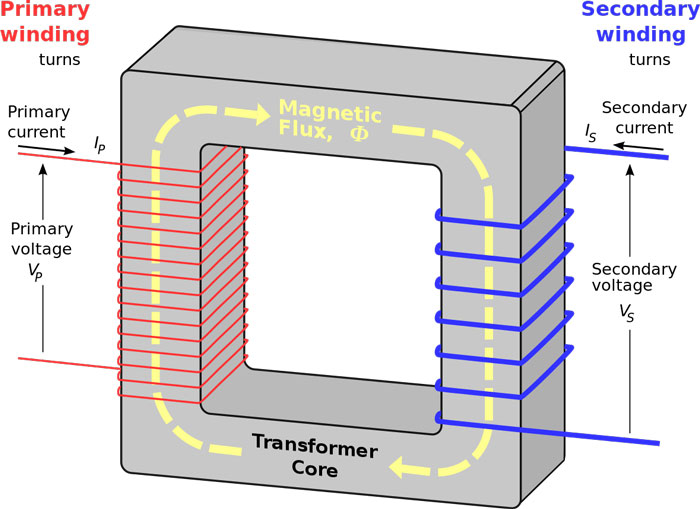Transformers are one of the most important electrical machines that you may be familiar with. They can be observed on poles that distribute electricity to houses and building in many nations. But in developed nations and densely populated cities, it is very hard to find them on local poles because they are buried in the ground. Let’s learn about these amazing machines.

## What Are Transformers?

Transformers are the electrical machines that are used to change the AC voltage, like in your power sockets, from one level to another. For example, if there is 220 volts (AC) in a power line and it needs to be converted into 110 volts (AC) then transformer will be used. Transformers can also be used to increase the voltage level (e.g. 110 volts to 220 volts).

## How Transformers Work?Transformers work on alternating current (AC) because they rely on the law of electromagnetic induction for functioning. In electromagnetic induction, when a magnetic field changes around an electrical conductor (wire), there will be a production of electrical current in that conductor. All the transformers rely on this principle for functioning.

A transformer is mainly made of three basic components; A core, primary winding, and secondary winding. The core is made of a material which enhances the magnetic field. Primary and secondary windings are the coils of wire that are wound on the core. These two winding are not connected together; only wound on the core separately.

When an alternating current is applied on primary winding, it will act as an electromagnet with changing magnetic field. The changing magnetic field will travel through the core and will pass through the secondary winding. Due to changing magnetic field, there will be the production of alternating current in the secondary winding.

So, how much voltage will be produced in secondary winding? Find out by reading turn ratio of transformers.

## Turn Ratio of Transformers

The production of voltage in secondary winding depends on a ratio, called turn ratio of a transformer. Turns ratio depends on the turns of wire wound on the primary and secondary winding.

For example: if there are 10 turns of wire on the primary winding and 40 turns on the secondary winding. Then, the turn ratio of the transformer will be 40 divided by 10 (40/10), which will be equal to 4. Now, multiply this turn ratio with voltage (AC) that is supplied to the primary winding. For example, if the applied voltage to the primary winding is 55 volts, then the voltage on secondary winding will be 55 × 4 = 220 volts.

The above-discussed transformer increases the voltage level from 55 volts to 220 volts, so it is called a step-up transformer. When a transformer decrease a voltage, suppose from 220 volts to 110 volts, it will be called a step-down transformer.

## UsesTransformers have played a vital role in the transmission of electricity over long distances. Prior to the transformer, transmission of electricity to hundreds of kilometers was very difficult and caused a lot of electricity loss. The loss was caused by heating-up of electrical conductors that carried the electricity. Because, when a current pass through a conductor it generates heat due to the resistance present in the conductors. By increasing the voltage of electricity, the amount of current can be reduced, which further reduces the waste of electricity and heating of conductors.

Today, power companies increasing the voltage (AC) on the conductors to transmit the electricity to large distances. When the electricity reaches to its consumers, for example to your house, it’s voltage is decreased (i.e. 220 volts or 110 volts) so that you can use it. Actually, there are a lot of transformers on the way, but the main purpose is same.

Here are a few more common uses of transformers:

• They are used in many household appliances to reduce the voltage to 12 or even low to be converted into DC so that the appliance can use it.
• These are used in sodium or neon street lamps to increase the voltage 11,000 volts to power the lamps.

## Facts

• The idea behind the invention of electrical transformers was given in 1880.
• Many nations increase the voltage to 500,000 volts (500KV) or more to transmit electricity to thousands of kilometers away.
• You can’t use direct current in a transformer, because direct current doesn’t produce changing magnetic field.